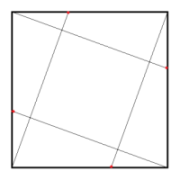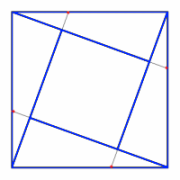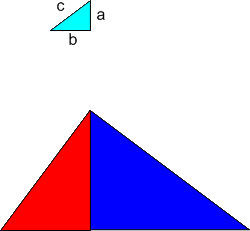#### You may also like### Doodles

Draw a 'doodle' - a closed intersecting curve drawn without taking pencil from paper. What can you prove about the intersections?### Russian Cubes

I want some cubes painted with three blue faces and three red faces. How many different cubes can be painted like that?### Picture Story

Can you see how this picture illustrates the formula for the sum of the first six cube numbers?

# Pythagoras Proofs

##### Age 14 to 16Challenge Level

Here are three different ideas which can lead to proofs of Pythagoras' Theorem. Can you make sense of them? Which proof do you find most "convincing"? Which do you find easiest to understand? Which would you find easiest to explain to someone else?

Firstly:

Draw a square and mark a point a fixed distance from each corner. Join from each of these points to the nearest corner on the opposite side as in the left hand drawing. Cut out the pieces marked in blue and rearrange them to make an L shape like the one on the right.Use your cutouts to prove Pythagoras' Theorem.

Secondly:

Move the slider to rotate a copy of the trapezium about the centre of the longest side of the blue triangle to make a square. What is the area of the square? From this formula for the area of this square derive a formula for the area of the trapezium. Now write down the area of the trapezium as the sum of the areas of the three right angled triangles. Use these results to give a proof of Pythagoras' Theorem explaining each step.

Thirdly:

Take any right-angled triangle and label its sides $a,b$ and $c$.Enlarge it by scale factor $a$ to make the red triangle and by scale factor $b$ to make the blue triangle.
Join the two enlargements together as shown.
Show that the two joined enlargements form an enlargement of the original triangle by scale factor $c$, and use this to prove Pythagoras' Theorem.